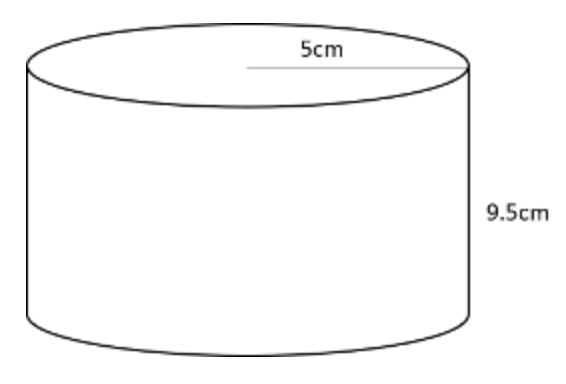# How do you find the Area of a Circle & the Surface Area of a Cylinder?

Finding the Area of a Circle is fairly simple if you use the correct formula. Finding the Surface Area of a Cylinder requires more complexity, as you have to break down the cylinder into it's smaller parts. Let's practice some problem sets together.## What is the Area of Circle?

Area is defined as the amount of space taken up by a two-dimential shape or surface. Area is measured by taking the total amount of1 unit by 1 unit squares that can fit within a two-dimensional shape or surface.

The area of a circle can be calculated by using the following formula:

## Area of a Circle

A = Area of Circle

π = 3.14 (rounded down) or 22/7

r = radius of the circle

#### Example Problem 1:

Find the area of a circle whose radius is 12 cm. Use 3.14 as π.

Step 1: Let's plug in the radius and π in the formula. The radius is 12 and π is 3.14.

Step 2: Simplify and write answer in unit squared.

So the area of a circle whose radius is 12 cm is 452.16 cm squared!

#### Example Problem 2:

Find the area of a circle whose diameter is 28 inches. Let's use 22/7 for π this time around.

Step 1: Find the radius. If the diameter is 28 inches then the radius is 14. it is half the length of the diameter.

Step 2: Let's plug in the radius and π in the formula. The radius is 12 and π is 22/7.

Step 3: Simply and calculate the Area!

So the area of a circle whose diameter is 28 inches is 616 inches squared!

## How is the area of a circle related to finding the surface area of a cylinder?

Surface area is the sum of the area of all surfaces on a three-dimensional figure. The best way to think of surface area is if you took a three-dimension figure and flattened out the figure to see all the faces. This is also called a net. Look at the following example using a cylinder:

In the example above, the net of a cylinder consists of two circles and a rectanglular shaped figure that is actually curved. You will need to find the area of each part and then add the sum to get the surface area. While the circles use the normal area formula of a circle, the curved rectangular shaped figure uses the circumference formula for its length. Recall, that the area of a rectangle is Area = Length X Height, or A = l x h

## How to find the surface area of a cylinder:

Area of the Circles   +   Area of Curved Rectangle  = Surface Area

#### Example Problem 3 - Cylinder:

Find the surface area of cylinder with a radius of 4 inches and a height of 6 inches. Use 3.14 as π

Step 1: Identify the radius and height.

r= 4 inches

h= 6 inches

Step 2: Substitute the values into the formula.

Step 3: Simplify.

SA = 2 ((3.14 * 16) + 2 (3.14) (4) (6)

SA = 2 (3.14 * 16) + 2 (75.36)

SA = 2 (50.24) + 2 (75.36)

SA = 100.48 + 150.72

SA = 251.2 inches squared.

So the surface area of a cylinder with a radius of 4 inches and a height of 6 inchesis 251.2 inches squared.

#### Example Problem 4 - Cylinder:

Find the surface area of this cylinder. Use 3.14 as π.

Step 1: Identify the radius and height.

r= 5cm and h=9.5cm

Step 2: Plug in the values into the formula.

Step 3: Simplify.

SA = 2 (3.14 * 25) + (2(3.14)(5))*(9.5)

SA = 2 (3.14 * 25) + 2*(15.7)*(9.5)

SA = 157 + 298.3

SA = 455.3 inches squared

So after adding up the area of the circles and area of the rectangle, the answer came 455.3 inches squared.